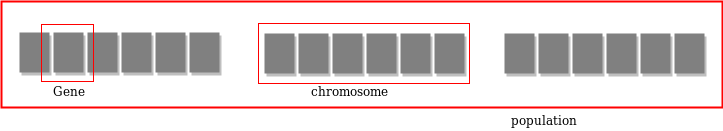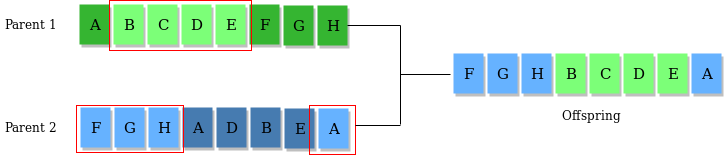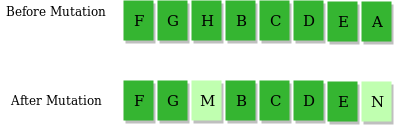Related Articles

# Genetic Algorithms

• Difficulty Level : Medium
• Last Updated : 27 Sep, 2021

Genetic Algorithms(GAs) are adaptive heuristic search algorithms that belong to the larger part of evolutionary algorithms. Genetic algorithms are based on the ideas of natural selection and genetics. These are intelligent exploitation of random search provided with historical data to direct the search into the region of better performance in solution space. They are commonly used to generate high-quality solutions for optimization problems and search problems.

Genetic algorithms simulate the process of natural selection which means those species who can adapt to changes in their environment are able to survive and reproduce and go to next generation. In simple words, they simulate “survival of the fittest” among individual of consecutive generation for solving a problem. Each generation consist of a population of individuals and each individual represents a point in search space and possible solution. Each individual is represented as a string of character/integer/float/bits. This string is analogous to the Chromosome.

Foundation of Genetic Algorithms

Genetic algorithms are based on an analogy with genetic structure and behavior of chromosomes of the population. Following is the foundation of GAs based on this analogy –

1. Individual in population compete for resources and mate
2. Those individuals who are successful (fittest) then mate to create more offspring than others
3. Genes from “fittest” parent propagate throughout the generation, that is sometimes parents create offspring which is better than either parent.
4. Thus each successive generation is more suited for their environment.

Search space

The population of individuals are maintained within search space. Each individual represents a solution in search space for given problem. Each individual is coded as a finite length vector (analogous to chromosome) of components. These variable components are analogous to Genes. Thus a chromosome (individual) is composed of several genes (variable components).Fitness Score

A Fitness Score is given to each individual which shows the ability of an individual to “compete”. The individual having optimal fitness score (or near optimal) are sought.

The GAs maintains the population of n individuals (chromosome/solutions) along with their fitness scores.The individuals having better fitness scores are given more chance to reproduce than others. The individuals with better fitness scores are selected who mate and produce better offspring by combining chromosomes of parents. The population size is static so the room has to be created for new arrivals. So, some individuals die and get replaced by new arrivals eventually creating new generation when all the mating opportunity of the old population is exhausted. It is hoped that over successive generations better solutions will arrive while least fit die.

Each new generation has on average more “better genes” than the individual (solution) of previous generations. Thus each new generations have better “partial solutions” than previous generations. Once the offsprings produced having no significant difference than offspring produced by previous populations, the population is converged. The algorithm is said to be converged to a set of solutions for the problem.

Operators of Genetic Algorithms

Once the initial generation is created, the algorithm evolve the generation using following operators –
1) Selection Operator: The idea is to give preference to the individuals with good fitness scores and allow them to pass there genes to successive generations.
2) Crossover Operator: This represents mating between individuals. Two individuals are selected using selection operator and crossover sites are chosen randomly. Then the genes at these crossover sites are exchanged thus creating a completely new individual (offspring). For example –3) Mutation Operator: The key idea is to insert random genes in offspring to maintain the diversity in the population to avoid premature convergence. For example –The whole algorithm can be summarized as –

```1) Randomly initialize populations p
2) Determine fitness of population
3) Until convergence repeat:
a) Select parents from population
b) Crossover and generate new population
c) Perform mutation on new population
d) Calculate fitness for new population```

Example problem and solution using Genetic Algorithms

Given a target string, the goal is to produce target string starting from a random string of the same length. In the following implementation, following analogies are made –

• Characters A-Z, a-z, 0-9, and other special symbols are considered as genes
• A string generated by these characters is considered as chromosome/solution/Individual

Fitness score is the number of characters which differ from characters in target string at a particular index. So individual having lower fitness value is given more preference.

## C++

 `// C++ program to create target string, starting from``// random string using Genetic Algorithm` `#include ``using` `namespace` `std;` `// Number of individuals in each generation``#define POPULATION_SIZE 100` `// Valid Genes``const` `string GENES = ``"abcdefghijklmnopqrstuvwxyzABCDEFGHIJKLMNOP"``\``"QRSTUVWXYZ 1234567890, .-;:_!\"#%&/()=?@\${[]}"``;` `// Target string to be generated``const` `string TARGET = ``"I love GeeksforGeeks"``;` `// Function to generate random numbers in given range``int` `random_num(``int` `start, ``int` `end)``{``    ``int` `range = (end-start)+1;``    ``int` `random_int = start+(``rand``()%range);``    ``return` `random_int;``}` `// Create random genes for mutation``char` `mutated_genes()``{``    ``int` `len = GENES.size();``    ``int` `r = random_num(0, len-1);``    ``return` `GENES[r];``}` `// create chromosome or string of genes``string create_gnome()``{``    ``int` `len = TARGET.size();``    ``string gnome = ``""``;``    ``for``(``int` `i = 0;ichromosome = chromosome;``    ``fitness = cal_fitness();``};` `// Perform mating and produce new offspring``Individual Individual::mate(Individual par2)``{``    ``// chromosome for offspring``    ``string child_chromosome = ``""``;` `    ``int` `len = chromosome.size();``    ``for``(``int` `i = 0;i population;``    ``bool` `found = ``false``;` `    ``// create initial population``    ``for``(``int` `i = 0;i new_generation;` `        ``// Perform Elitism, that mean 10% of fittest population``        ``// goes to the next generation``        ``int` `s = (10*POPULATION_SIZE)/100;``        ``for``(``int` `i = 0;i

## Python

 `# Python3 program to create target string, starting from``# random string using Genetic Algorithm` `import` `random` `# Number of individuals in each generation``POPULATION_SIZE ``=` `100` `# Valid genes``GENES ``=` `'''abcdefghijklmnopqrstuvwxyzABCDEFGHIJKLMNOP``QRSTUVWXYZ 1234567890, .-;:_!"#%&/()=?@\${[]}'''` `# Target string to be generated``TARGET ``=` `"I love GeeksforGeeks"` `class` `Individual(``object``):``    ``'''``    ``Class representing individual in population``    ``'''``    ``def` `__init__(``self``, chromosome):``        ``self``.chromosome ``=` `chromosome``        ``self``.fitness ``=` `self``.cal_fitness()` `    ``@classmethod``    ``def` `mutated_genes(``self``):``        ``'''``        ``create random genes for mutation``        ``'''``        ``global` `GENES``        ``gene ``=` `random.choice(GENES)``        ``return` `gene` `    ``@classmethod``    ``def` `create_gnome(``self``):``        ``'''``        ``create chromosome or string of genes``        ``'''``        ``global` `TARGET``        ``gnome_len ``=` `len``(TARGET)``        ``return` `[``self``.mutated_genes() ``for` `_ ``in` `range``(gnome_len)]` `    ``def` `mate(``self``, par2):``        ``'''``        ``Perform mating and produce new offspring``        ``'''` `        ``# chromosome for offspring``        ``child_chromosome ``=` `[]``        ``for` `gp1, gp2 ``in` `zip``(``self``.chromosome, par2.chromosome):   ` `            ``# random probability ``            ``prob ``=` `random.random()` `            ``# if prob is less than 0.45, insert gene``            ``# from parent 1``            ``if` `prob < ``0.45``:``                ``child_chromosome.append(gp1)` `            ``# if prob is between 0.45 and 0.90, insert``            ``# gene from parent 2``            ``elif` `prob < ``0.90``:``                ``child_chromosome.append(gp2)` `            ``# otherwise insert random gene(mutate),``            ``# for maintaining diversity``            ``else``:``                ``child_chromosome.append(``self``.mutated_genes())` `        ``# create new Individual(offspring) using``        ``# generated chromosome for offspring``        ``return` `Individual(child_chromosome)` `    ``def` `cal_fitness(``self``):``        ``'''``        ``Calculate fittness score, it is the number of``        ``characters in string which differ from target``        ``string.``        ``'''``        ``global` `TARGET``        ``fitness ``=` `0``        ``for` `gs, gt ``in` `zip``(``self``.chromosome, TARGET):``            ``if` `gs !``=` `gt: fitness``+``=` `1``        ``return` `fitness` `# Driver code``def` `main():``    ``global` `POPULATION_SIZE` `    ``#current generation``    ``generation ``=` `1` `    ``found ``=` `False``    ``population ``=` `[]` `    ``# create initial population``    ``for` `_ ``in` `range``(POPULATION_SIZE):``                ``gnome ``=` `Individual.create_gnome()``                ``population.append(Individual(gnome))` `    ``while` `not` `found:` `        ``# sort the population in increasing order of fitness score``        ``population ``=` `sorted``(population, key ``=` `lambda` `x:x.fitness)` `        ``# if the individual having lowest fitness score ie.``        ``# 0 then we know that we have reached to the target``        ``# and break the loop``        ``if` `population[``0``].fitness <``=` `0``:``            ``found ``=` `True``            ``break` `        ``# Otherwise generate new offsprings for new generation``        ``new_generation ``=` `[]` `        ``# Perform Elitism, that mean 10% of fittest population``        ``# goes to the next generation``        ``s ``=` `int``((``10``*``POPULATION_SIZE)``/``100``)``        ``new_generation.extend(population[:s])` `        ``# From 50% of fittest population, Individuals``        ``# will mate to produce offspring``        ``s ``=` `int``((``90``*``POPULATION_SIZE)``/``100``)``        ``for` `_ ``in` `range``(s):``            ``parent1 ``=` `random.choice(population[:``50``])``            ``parent2 ``=` `random.choice(population[:``50``])``            ``child ``=` `parent1.mate(parent2)``            ``new_generation.append(child)` `        ``population ``=` `new_generation` `        ``print``(``"Generation: {}\tString: {}\tFitness: {}"``.\``              ``format``(generation,``              ``"".join(population[``0``].chromosome),``              ``population[``0``].fitness))` `        ``generation ``+``=` `1` `    ` `    ``print``(``"Generation: {}\tString: {}\tFitness: {}"``.\``          ``format``(generation,``          ``"".join(population[``0``].chromosome),``          ``population[``0``].fitness))` `if` `__name__ ``=``=` `'__main__'``:``    ``main()`

Output:

```Generation: 1    String: tO{"-?=jH[k8=B4]Oe@}    Fitness: 18
Generation: 2    String: tO{"-?=jH[k8=B4]Oe@}    Fitness: 18
Generation: 3    String: .#lRWf9k_Ifslw #O\$k_    Fitness: 17
Generation: 4    String: .-1Rq?9mHqk3Wo]3rek_    Fitness: 16
Generation: 5    String: .-1Rq?9mHqk3Wo]3rek_    Fitness: 16
Generation: 6    String: A#ldW) #lIkslw cVek)    Fitness: 14
Generation: 7    String: A#ldW) #lIkslw cVek)    Fitness: 14
Generation: 8    String: (, o x _x%Rs=, 6Peek3   Fitness: 13
.
.
.
Generation: 29    String: I lope Geeks#o, Geeks   Fitness: 3
Generation: 30    String: I loMe GeeksfoBGeeks    Fitness: 2
Generation: 31    String: I love Geeksfo0Geeks    Fitness: 1
Generation: 32    String: I love Geeksfo0Geeks    Fitness: 1
Generation: 33    String: I love Geeksfo0Geeks    Fitness: 1
Generation: 34    String: I love GeeksforGeeks    Fitness: 0```

As we can see from the output, our algorithm sometimes stuck at a local optimum solution, this can be further improved by updating fitness score calculation algorithm or by tweaking mutation and crossover operators.

Why use Genetic Algorithms

• They are Robust
• Provide optimisation over large space state.
• Unlike traditional AI, they do not break on slight change in input or presence of noise

Application of Genetic Algorithms

Genetic algorithms have many applications, some of them are –

• Recurrent Neural Network
• Mutation testing
• Code breaking
• Filtering and signal processing
• Learning fuzzy rule base etc

References
https://en.wikipedia.org/wiki/List_of_genetic_algorithm_applications
https://en.wikipedia.org/wiki/Genetic_algorithm
https://www.doc.ic.ac.uk/~nd/surprise_96/journal/vol1/hmw/article1.html
This article is contributed by Atul Kumar. If you like GeeksforGeeks and would like to contribute, you can also write an article using write.geeksforgeeks.org or mail your article to review-team@geeksforgeeks.org. See your article appearing on the GeeksforGeeks main page and help other Geeks.